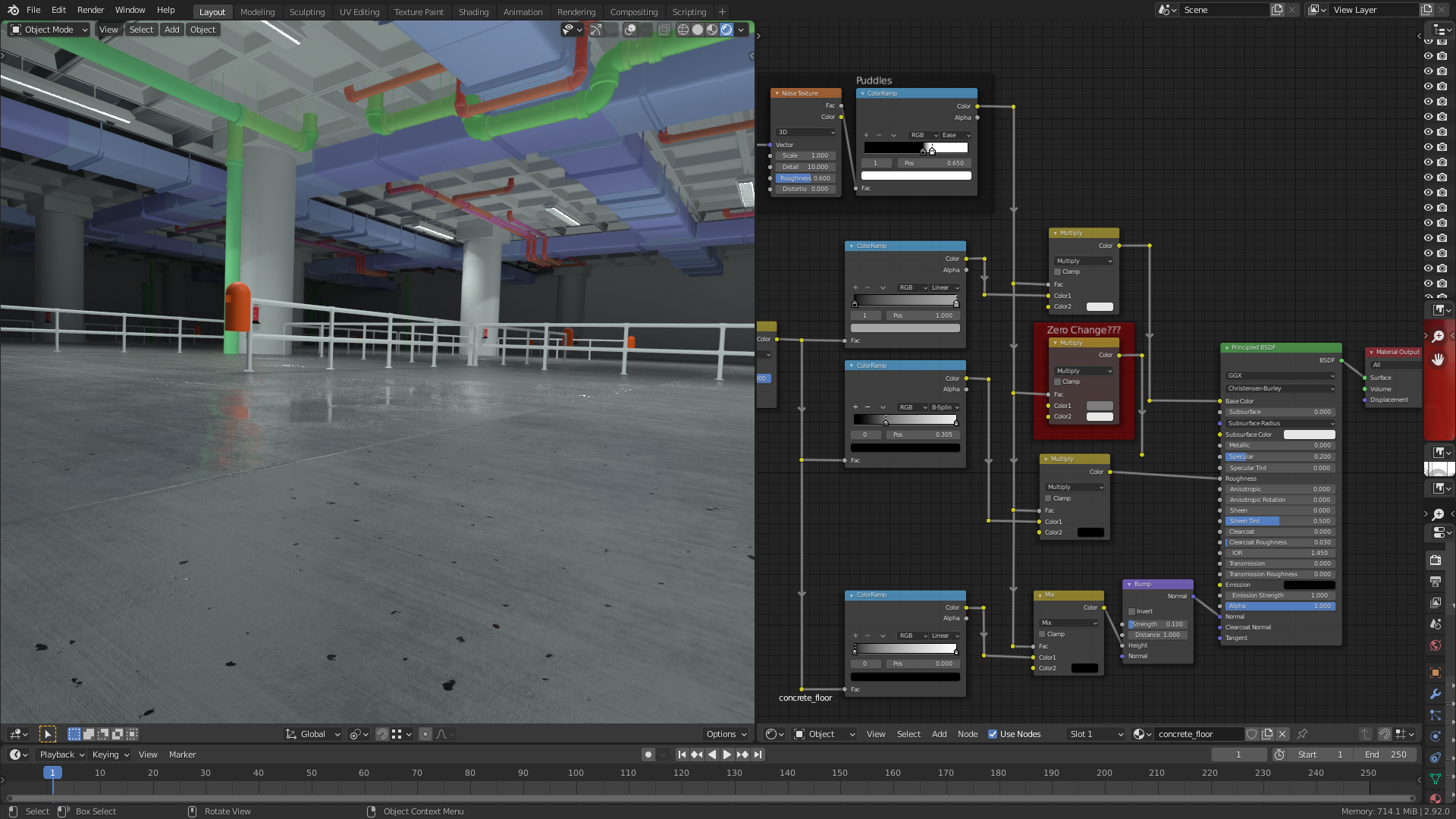2 answers · asked @ video mark 4:40 · Lesson: Adding Puddles to the Floor Material · Course: Create Realistic Industrial Environments with Blender and Eevee

## Realistic Industrial Environments Course: "Principled BSDF" Node's "Specular" Socket Input without Effect?theluthier In the node setup for the puddles the "Specular" socket input of the "Principled BSDF" node doesn't seem to have any effect on the shading:

1) With Connected "Specular" Socket2) With Unconnected "Specular" SocketMy explanation:

1) The "Math Node" in the red frame which is set to "Multiply" has grey as first color which has the same value for R=G=B= 0.2 als the unconnected "Specular" socket of the "Principled BSDF" node. The second color of the mentioned "Math Node" is pure white (R=G=B= 1).

2) The "Math Node" in "Multiply" mode calculates its output like this:

(with "color1" being the top socket and "color2" being the bottom socket; the following calculation has to be done for R, G and B of color1 and color2  separately; for the sake of simplicity, I didn't use any indices for these three base colors since they have the same values for pure white (1) and any tone of grey (0 < grey value < 1))

output = color1  * ((1-fac) + (fac*color2))

So, if fac is 0 (=black = no puddle) we get 0.2 as "Specular" value which is the same value as the unconnected "Specular" input socket of the "Principled BSDF" node.

If fac is 1 (=white = 100% puddle), color2 (R=G=B= 1) is multiplied with color1 so that the new values for R=G=B is 0.2 * 1 = 0.2. Nothing change again compared with the unconnected "Specular" input socket of the "Principled BSDF" node.

Let's finally take a value in between like for example fac = 0.5:

output = color1 * ((1-0.5) + (0.5 * color2))

= color1 * (0.5 + 0.5 * color2)

With R=G=B= 0.2 for color1 and R=G=B= 1 for color2, we get:

output = 0.2 * (0.5 + 0.5 *1)

= 0.2 * 1

= 0.2

So, in all three cases above, the connected  "Specular" input socket of the "Principled BSDF" node has the same value as the unconnected socket.

I think, the "Puddle Effect" comes only from the three other inputs:

1) A darker tone of grey for the "Base Color"

2) A "Roughness" value of 0 (= black)

3) A "Bump" value 0 (= black)

But the "Specularity" isn't raised at all.